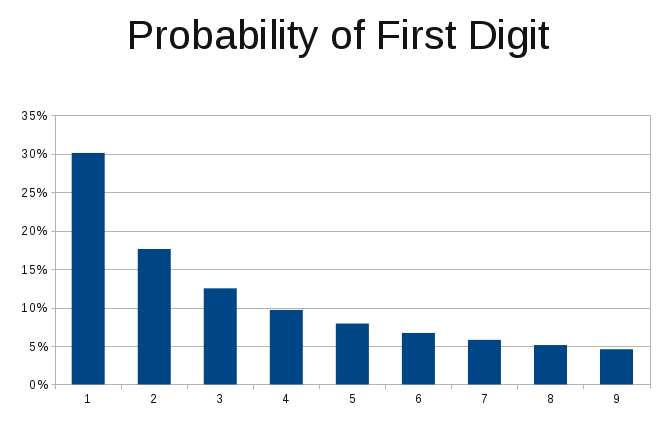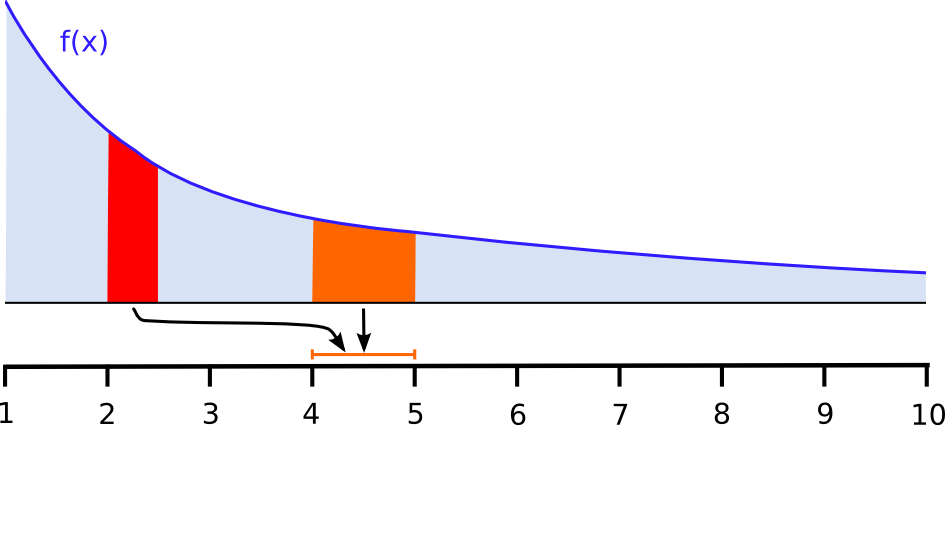# Detecting Fraud Using Benford’s Law: Mathematical Details

When people fabricate numbers for fraudulent purposes they often fail to take Benford’s Law into account, making it possible to detect the fraud.  This article is a supplement to my article “Detecting Fraud Using Benford’s Law” (if the link doesn’t take you directly to the right page, it is PDF page number 69 or printed page number 67) from the Summer 2015 issue of Criminal Justice.

Benford’s Law says that naturally occurring numbers that span several orders of magnitude (i.e., differing numbers of digits, or differing powers of 10 when written in scientific notation like 3.15 x 102) should start with “1” 30.1% of the time, and they should start with “9” only 4.6% of the time.  The probability of each leading digit is given in this chart (click to enlarge):Someone who attempts to commit fraud by fabricating numbers (e.g., fake invoices or accounting entries) without knowing Benford’s Law will probably generate numbers that don’t have the expected probability distribution.  They might, for example, assume that numbers starting with “1” should have the same probability as numbers starting with any other digit, resulting in their fraudulent numbers looking very suspicious to someone who knows Benford’s Law.

The Criminal Justice article details the history of Benford’s Law and explains when Benford’s Law is expected to be applicable.  What I’ll add here is more mathematical detail on how the probability of a particular leading digit, or sequence of digits, can be computed.

The key assumption behind Benford’s Law is scale invariance, meaning that things shouldn’t change if we switch to a different unit of measure.  If we convert a large set of monetary values from dollars to yen, or pesos, or any other currency (real or concocted), the percentage of values starting with a particular digit should stay (approximately) the same.  Suppose we convert from dollars to a currency that is worth half as much.  An item that costs $1 will cost 2 units of the new currency. An item that costs$1.99 will cost 3.98 units of the new currency.  Likewise, $1000 becomes 2000 units of the new currency, and$1999 becomes 3998 units of the new currency.  So the probability of a number starting with “1” has to equal the sum of the probabilities of a numbers starting with “2” or “3” if the probability of a particular digit will remain unchanged by switching currencies.  The probabilities from the bar chart above behave as expected (30.1% = 17.6% + 12.5%).

To prove that scale invariance leads to the probabilities predicted by Benford’s Law, start by converting all possible numbers to scientific notation (e.g. 315 is written as 3.15 x 102) and realize that the power of 10 doesn’t matter when our only concern is the probability of a certain leading digit.  So all numbers map to the interval [1,10) as shown in this figure:Next, assume there is some function, f(x), that gives the probability of each possible set of leading digits (technically a probability density function), so f(4.25) accounts for the probability of finding a value to be 0.0425, 0.425, 4.25, 42.5, 425, 4250, etc..  Our goal is to find f(x).  This graph illustrates the constraint that scale invariance puts on f(x):The area under the f(x) curve between x=2 and x=2.5, shown in red, must equal the area between x=3 and x=4, shown in orange, because a change in scale that multiplies all values by 2 will map the values from the red region into the orange region.  Such relationships between areas under various parts of the curve must be satisfied for any change of scale, not just a factor of two.

Finally, let’s get into the gory math and prove Benford’s Law (warning: calculus!).  The probability, P(D), of a number starting with digit D is the area under the f(x) curve from D to D+1:$P(D) = \int_D^{D+1} f(x) \,dx$

Assuming that scale invariance holds, the probability has to stay the same if we change scale such that all values are multiplied by β:$P(D) = \int_{\beta D}^{\beta (D+1)} f(x) \,dx$

The equation above must be true for any β, so the derivative with respect to β must be zero:$\frac{\partial}{\partial \beta} P(D) = 0 \ \ \ \Rightarrow\ \ \ (D + 1) f\left(\beta(D + 1)\right) - D f(\beta D) = 0$

The equation above is satisfied if f(x)=c/x, where c is a constant.  The total area under the f(x) curve must be 1 because it is the probability that a number will start with any possible set of digits, so that determines the value of c to be 1/ln(10), i.e. 1 over the natural logarithm of 10:$\int_1^{10} f(x) \,dx = 1 \ \ \ \Rightarrow\ \ \ f(x) = \frac{1}{x \ln(10)}$

Finally, plug f(x) into our first equation and integrate to get a result in terms of base-10 logarithms:$P(D) = \frac{\ln(D+1) - \ln(D)}{\ln(10)} = \log_{10}(D + 1) - \log_{10}(D)$

Knowing f(x), we can compute the probability of finding a number with any sequence of initial digits.  To find the probability of starting with 2 we integrated from 2 to 3.  To find the probability of starting with the two digits 24, we integrate f(x) from 2.4 to 2.5.  To find the probability of starting with the three digits 247, we integrate f(x) from 2.47 to 2.48.  The general equation for two leading digits, D1D2, is:$P(D_1D_2) = \log_{10}(D_1.D_2 + 0.1) - \log_{10}(D_1.D_2)$

Which is equivalent to:$P(D_1D_2) = \log_{10}(D_1D_2 + 1) - \log_{10}(D_1D_2)$

For example, the probability of a number starting with “2” followed by “4” is log10(25)-log10(24) = 1.77%.

Similarly, the equation for three leading digits, D1D2D3, is:$P(D_1D_2D_3) = \log_{10}(D_1D_2D_3 + 1) - \log_{10}(D_1D_2D_3)$